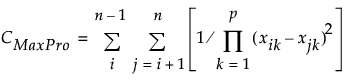Design of Experiments Guide > Space-Filling Designs > Fast Flexible Filling Designs
Publication date: 04/12/2021

# Fast Flexible Filling Designs

The algorithms for Fast Flexible Filling designs begin by generating a large number of random points within the specified design region. These points are then clustered using a Fast Ward algorithm into a number of clusters that equals the Number of Runs that you specified.

The final design points can be obtained by using the default MaxPro (maximum projection) optimality criterion or by selecting the Centroid criterion.

Note: If you have Categorical factors or factor constraints, then Fast Flexible Filling is the only space filling design Method available.

## FFF Optimality Criterion

The Space Filling Design red triangle menu contains optimality criteria under FFF Optimality Criterion.

MaxPro

For p factors and n equal to the specified Number of Runs, the MaxPro criterion strives to find points in the clusters that minimize the following criterion:The MaxPro criterion maximizes the product of the distances between potential design points in a way that involves all factors. This supports the goal of providing good space-filling properties on projections of factors. See Joseph et al. (2015). The Max Pro option is the default.

Centroid

This method places a design point at the centroid of each cluster. It has the property that the average distance from an arbitrary point in the design space to its closest neighboring design point is smaller than for other designs.

Note: You can set a preference to always use a given optimality criterion. Select File > Preferences > Platforms > DOE. Select FFF Optimality Criterion and select your preferred criterion.

### Categorical Factors

When you have categorical factors, the algorithm proceeds as follows:

The total number of design points is balanced across the total number of combinations of levels of the categorical factors. Suppose that there are m combinations of levels and that k design points are allocated to each of these.

A large number of points within the design space defined by the continuous variables is generated. These are grouped into k primary clusters.

Each of the k primary clusters of points is further clustered into m sub-clusters.

Within each primary cluster, a design point is calculated for each of the m sub-clusters using the specified FFF optimality criterion.

For each of the k primary clusters, one of the m combinations of levels is randomly assigned to each of the m sub-cluster design points. This yields a total of km design points.

For each of the k primary clusters, a design point is chosen for each of the m combinations of levels according to the MaxPro criterion, where points having the same level of a categorical factor are multiplied by the MaxPro Categorical Weight. (For a description of MaxPro Categorical Weight, see Space Filling Design Options.) This process continues through all k primary clusters 10 times, or until no improvement is found by changing the given design points.

Want more information? Have questions? Get answers in the JMP User Community (community.jmp.com).